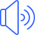multiply

المضارع او المصدرmultiplied

الماضيmultiplied

التصريف الثالثmultiplying

صيغة الـ ing

# استخدامات الفعل multiply مع جميع الازمنة الانجليزية

Simple Present Tense

# زمن المضارع البسيط

I multiply
You multiply
He multiplies
She multiplies
It multiplies
We multiply
You multiply
They multiply

لا يفوتك تحميل تطبيقنا المجاني الشامل والذي سوف يغنيك عن كل شي حيث سوف يبدء معك من مستوى الاحرف الانجليزية ويستمر معك الى مستوى الاحتراف بأذن الله مع اختبارات تفاعلية متعددة وشرح مفصل لكل صغيرة وكبيرة علما ان جميع الدروس والمقاطع الصوتية تعمل بدون الحاجة الى الانترنت. ولاتنسى تقيم التطبيق اذا كنت بالفعل حملته على جهازك

تطبيق الايفون تطبيق الاندرويد

Simple Past Tense

# زمن الماضي البسيط

I multiplied
You multiplied
He multiplied
She multiplied
It multiplied
We multiplied
You multiplied
They multiplied

Simple Future Tense

# زمن المستقبل البسيط

I will multiply
You will multiply
He will multiply
She will multiply
It will multiply
We will multiply
You will multiply
They will multiply

Present Perfect Tense

# زمن المضارع التام

I have multiplied
You have multiplied
He has multiplied
She has multiplied
It has multiplied
We have multiplied
You have multiplied
They have multiplied

Past Perfect Tense

# زمن الماضي التام

Future Perfect Tense

# زمن المستقبل التام

I will have multiplied
You will have multiplied
He will have multiplied
She will have multiplied
It will have multiplied
We will have multiplied
You will have multiplied
They will have multiplied

Present Continuous Tense

# زمن المضارع المستمر

I am multiplying
You are multiplying
He is multiplying
She is multiplying
It is multiplying
We are multiplying
You are multiplying
They are multiplying

Past Continuous Tense

# زمن الماضي المستمر

I was multiplying
You were multiplying
He was multiplying
She was multiplying
It was multiplying
We were multiplying
You were multiplying
They were multiplying

Future Continuous Tense

# زمن المستقبل المستمر

I will be multiplying
You will be multiplying
He will be multiplying
She will be multiplying
It will be multiplying
We will be multiplying
You will be multiplying
They will be multiplying

Present Perfect Continuous Tense

# زمن المضارع التام المستمر

I have been multiplying
You have been multiplying
He has been multiplying
She has been multiplying
It has been multiplying
We have been multiplying
You have been multiplying
They have been multiplying

Past Perfect Continuous Tense

# زمن الماضي التام المستمر

Future Perfect Continuous Tense

# زمن المستقبل التام المستمر

I will have been multiplying
You will have been multiplying
He will have been multiplying
She will have been multiplying
It will have been multiplying
We will have been multiplying
You will have been multiplying
They will have been multiplying

لكي تتابع دروسنا الجديدة بالصوت والصورة والشرح المفصل انصحك بالاشتراك في قناتنا على اليوتيوب

او انشر الصفحة على مواقع التواصل الاجتماعي

### كلمات دالة

multiply تصريف فعل

multiplied تصريف الفعل

multiplied تصريف فعل

multiply تصريف الفعل

multiply ترجمة و معنى كلمة

multiply معنى الفعل

multiply ترجمة الفعل